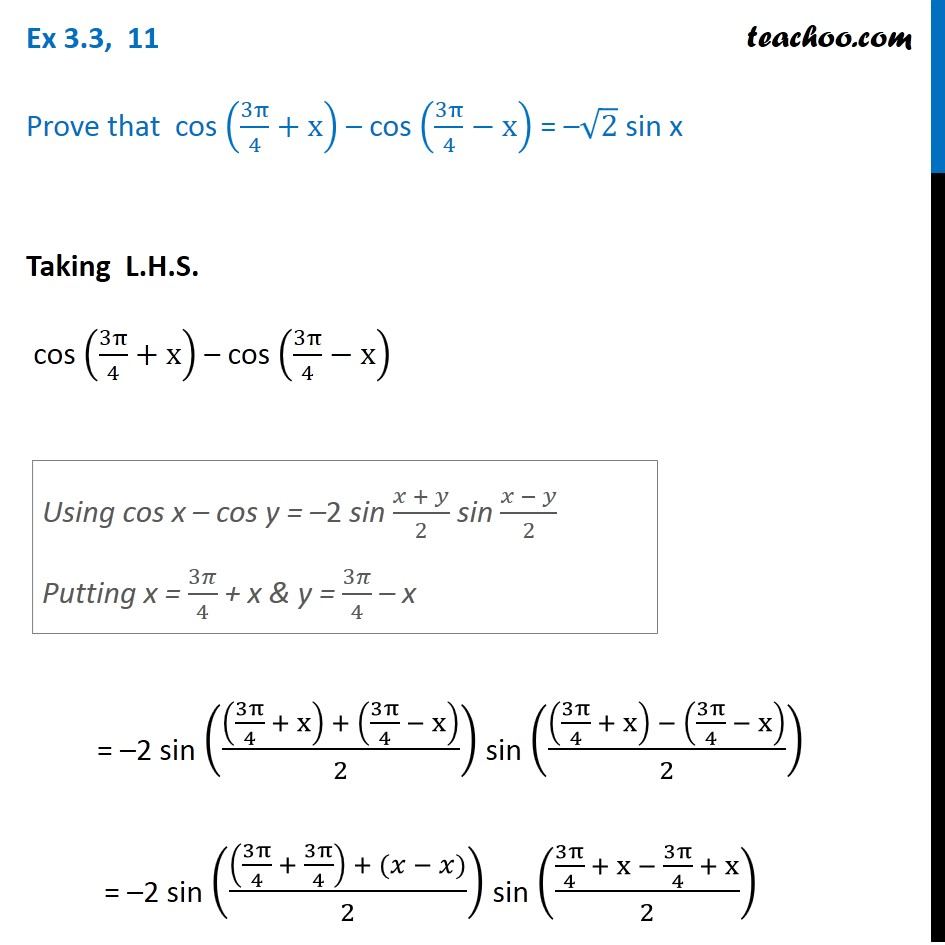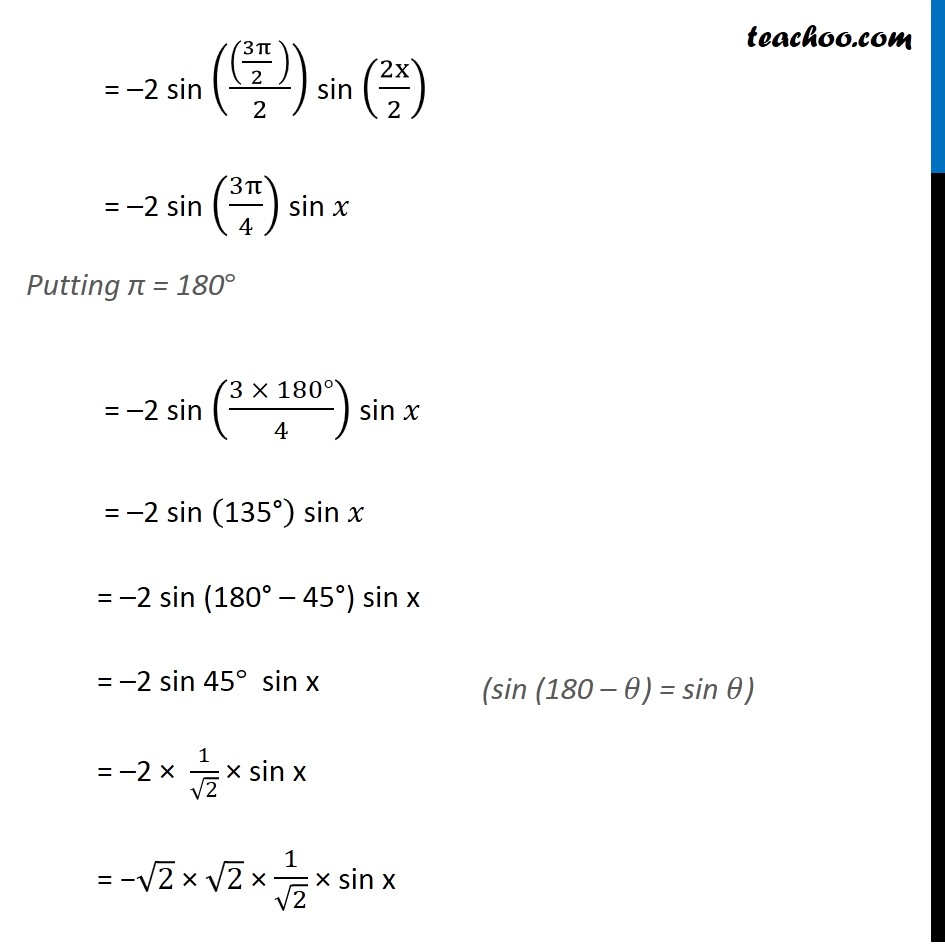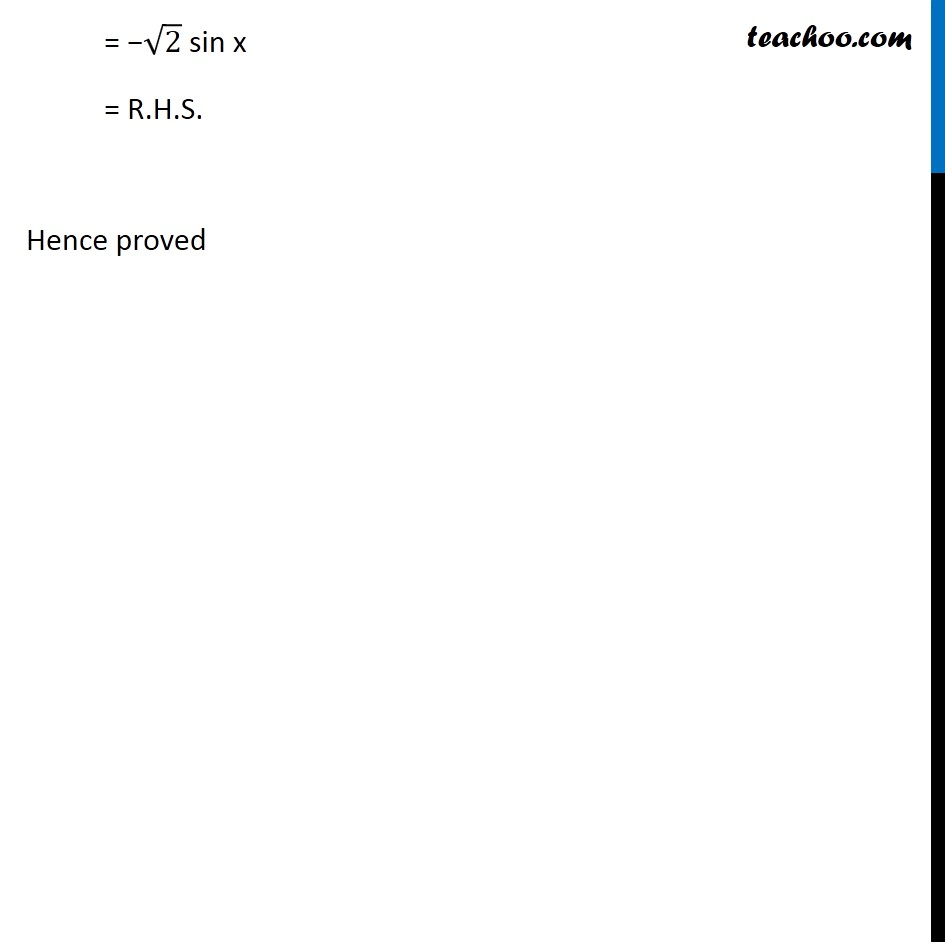Learn All Concepts of Chapter 2 Class 11 Relations and Function - FREE. Check - Trigonometry Class 11 - All Concepts1. Chapter 3 Class 11 Trigonometric Functions
2. Serial order wise
3. Ex 3.3

Transcript

Ex 3.3, 11 Prove that cos (3π/4+x) – cos (3π/4−x) = –√2 sin x Taking L.H.S. cos (3π/4+x) – cos (3π/4−x) = –2 sin (((3π/4 + x) + (3π/4 − x))/2) sin (((3π/4 + x) − (3π/4 − x))/2) = –2 sin (((3π/4 + 3π/4) + (𝑥 − 𝑥))/2) sin ((3π/4 + x − 3π/4 + x)/2) Using cos x – cos y = –2 sin (𝑥 + 𝑦)/2 sin (𝑥 − 𝑦)/2 Putting x = 3𝜋/4 + x & y = 3𝜋/4 – x = –2 sin (((3π/2 ))/2) sin (2x/2) = –2 sin (3π/4) sin 𝑥 Putting π = 180° = –2 sin ((3 × 180°)/4) sin 𝑥 = –2 sin ("135°" ) sin 𝑥 = –2 sin (180"°" – 45"°") sin x = –2 sin 45° sin x = –2 × 1/√2 × sin x = −√2 × √2 × 1/√2 × sin x (sin (180 – 𝜃) = sin 𝜃) = −√2 sin x = R.H.S. Hence proved

Ex 3.3

About the AuthorDavneet Singh
Davneet Singh is a graduate from Indian Institute of Technology, Kanpur. He has been teaching from the past 9 years. He provides courses for Maths and Science at Teachoo.